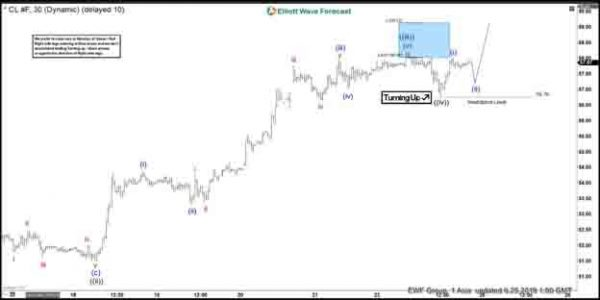Oil (CL_F) rally from June 5, 2019 low is unfolding as an impulse Elliott Wave structure where wave 3 is currently in progress. In the chart below, we can see wave ((ii)) of 3 ended at 51.5, wave ((iii)) of 3 ended at 58.22, and wave ((iv)) of 3 ended at 56.76. Wave ((iii)) shows an extension which is typical in an impulsive structure. The internal of wave ((iii)) unfolded as an impulse of lesser degree. Up from 51.5, wave (i) ended at 54.38, wave (ii) ended at 53.28, wave (iii) ended at 57.98, wave (iv) ended at 57.04, and wave (v) ended at 58.22.

Near term, while pullback stays above wave ((iv)) at 56.76, expect CL_F to extend higher. However, a break above wave ((iii)) at 58.22 is still required to avoid a double correction in wave ((iv)). If Oil breaks below 56.76 instead, then it is doing a double correction and should find the next support at 55.7 – 56.6 for further upside or 3 waves bounce at least. We don’t like selling Oil and expect dips to continue finding support in 3, 7, or 11 swing for further upside.

Oil (CL_F) 1 Hour Elliott Wave Chart